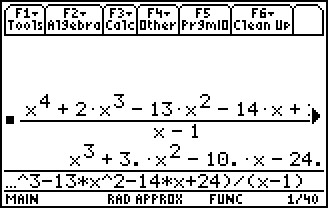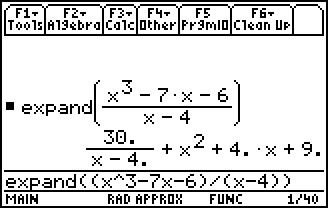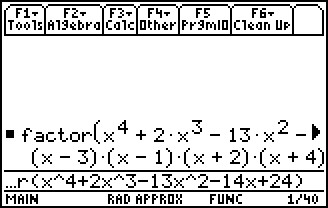# Activities

••• ##### Subject Area

• Math: Precalculus: Polynomial, Power, and Rational Functions

• ##### Author9-12

20 Minutes

• ##### Device
• TI-89 / TI-89 Titanium
• ##### Report an Issue

Polly, Want Some Division?#### Activity Overview

Students use polynomial calculations to determine quotients and remainders when performing polynomial division.

#### Key Steps

•In this activity, students will explore problems involving division of polynomials. Through this process students will develop an understanding of the Remainder Theorem, which is useful in identifying zeros of functions and in evaluating function values.

•Students will divide polynomials and use the Expand command to view the remainder if one exists.

•The Factor command is used in developing an understanding of the results of polynomial division.

Results of division are explored graphically to help students gain a deeper understanding of the connection between dividends, divisors, and quotients.